# Selina Solutions Concise Maths Class 7 Chapter 6: Ratio and Proportion (Including Sharing in a Ratio) Exercise 6B

Selina Solutions Concise Maths Class 7 Chapter 6 Ratio and Proportion (Including Sharing in a Ratio) Exercise 6B help students understand the concept of proportion and continued proportion. When the ratio between first and second quantity is equal to the third and fourth quantities, then they are said to be in proportion. Students can solve the exercise wise problems by referring to the solutions PDF to gain a better hold on these concepts. Selina Solutions Concise Maths Class 7 Chapter 6 Ratio and Proportion (Including Sharing in a Ratio) Exercise 6B, PDF links are available here.

## Selina Solutions Concise Maths Class 7 Chapter 6: Ratio and Proportion (Including Sharing in a Ratio) Exercise 6B Download PDF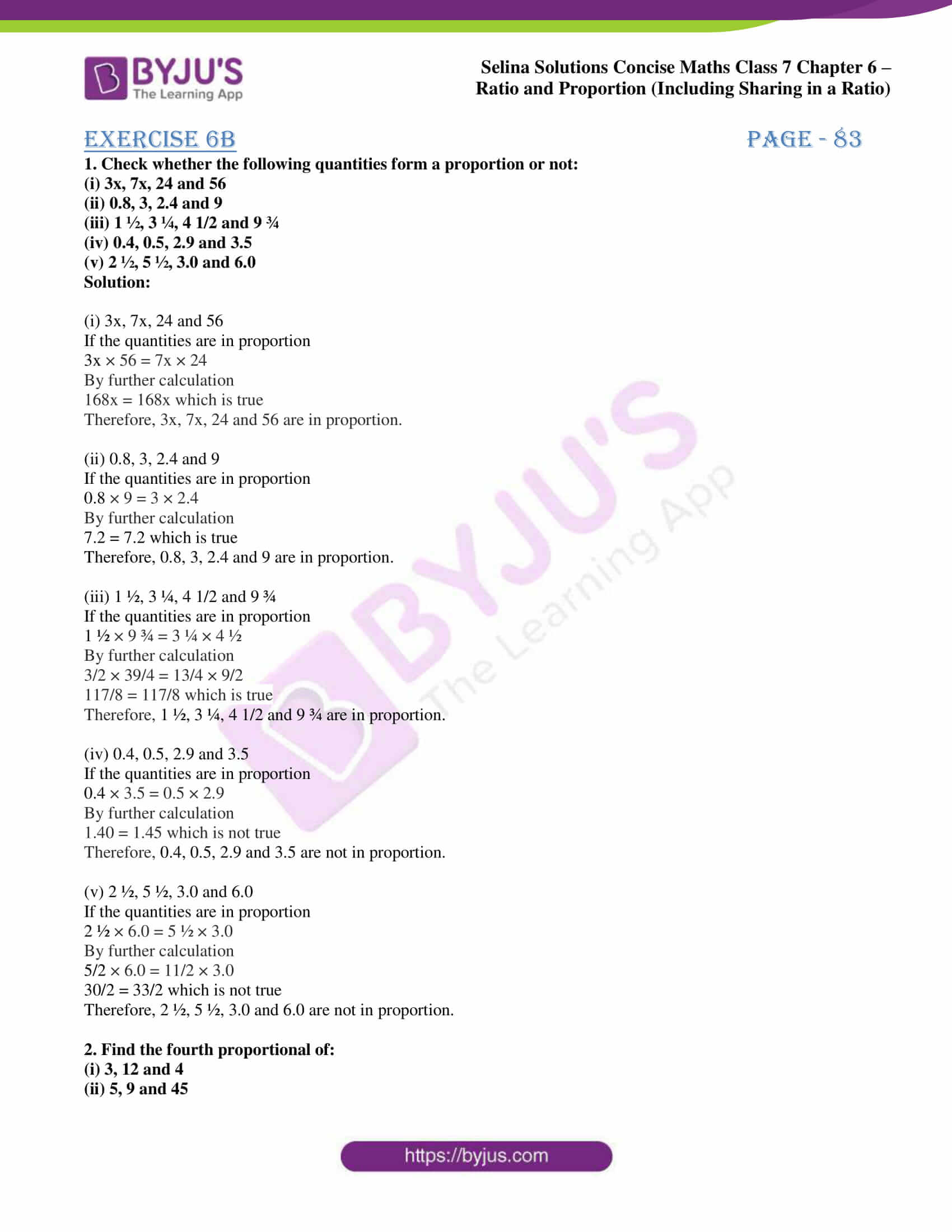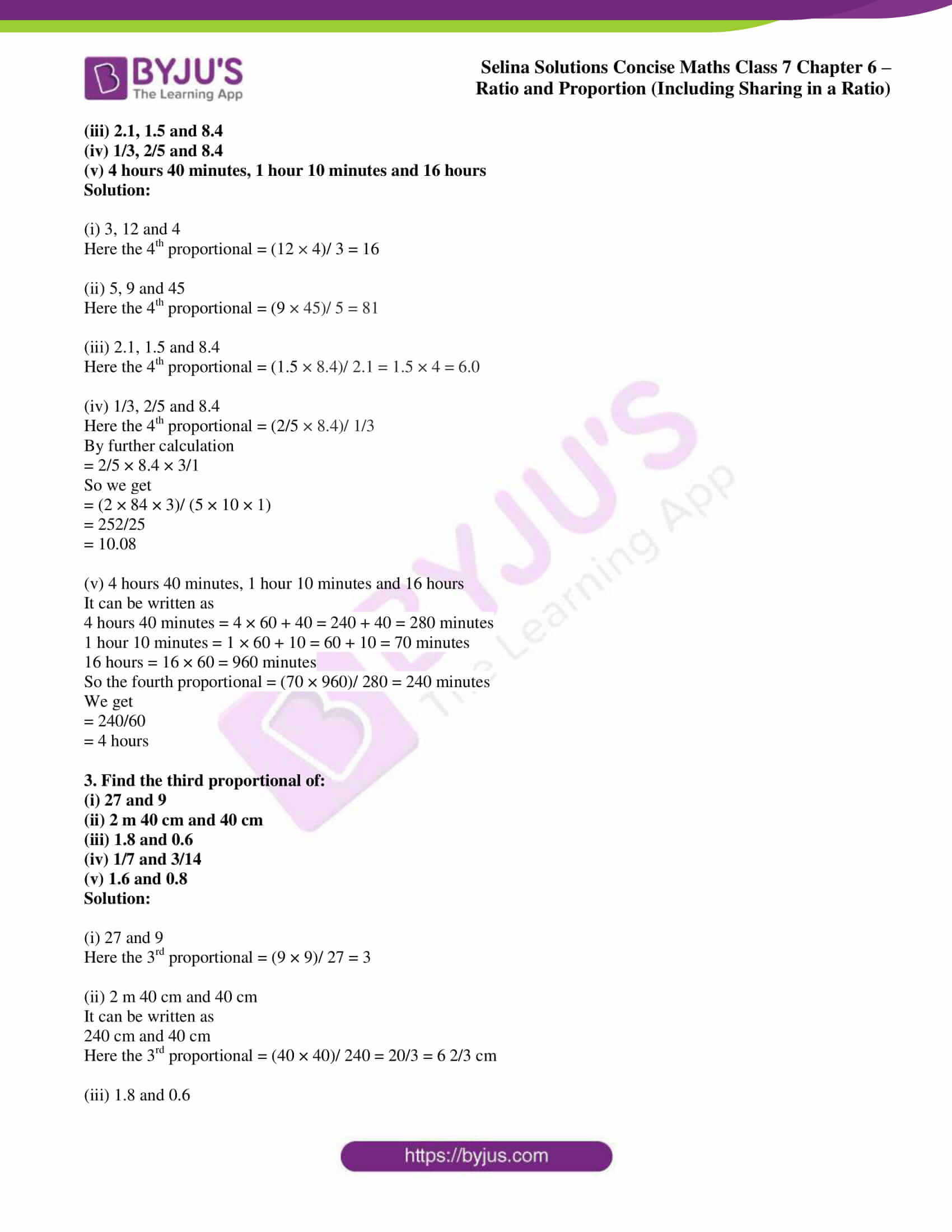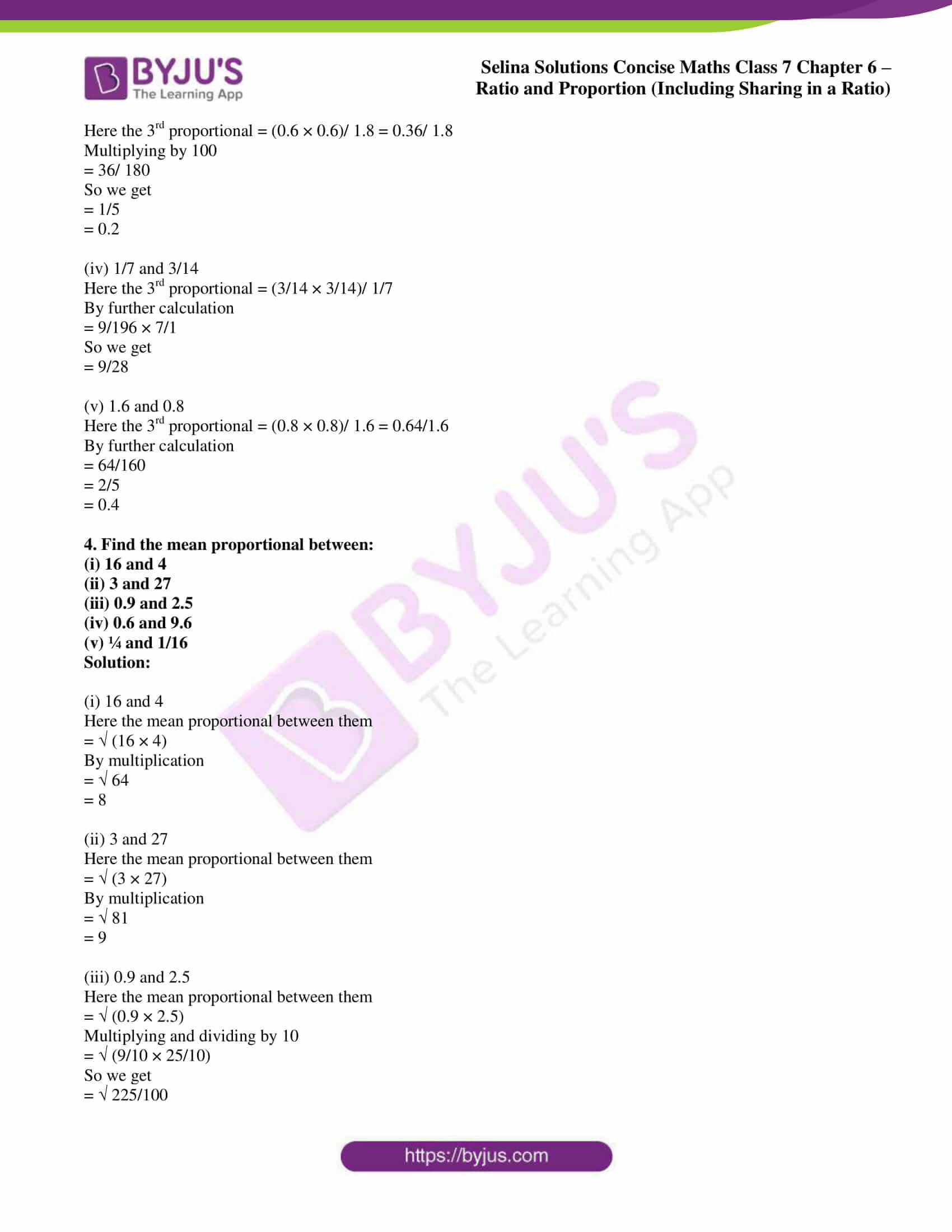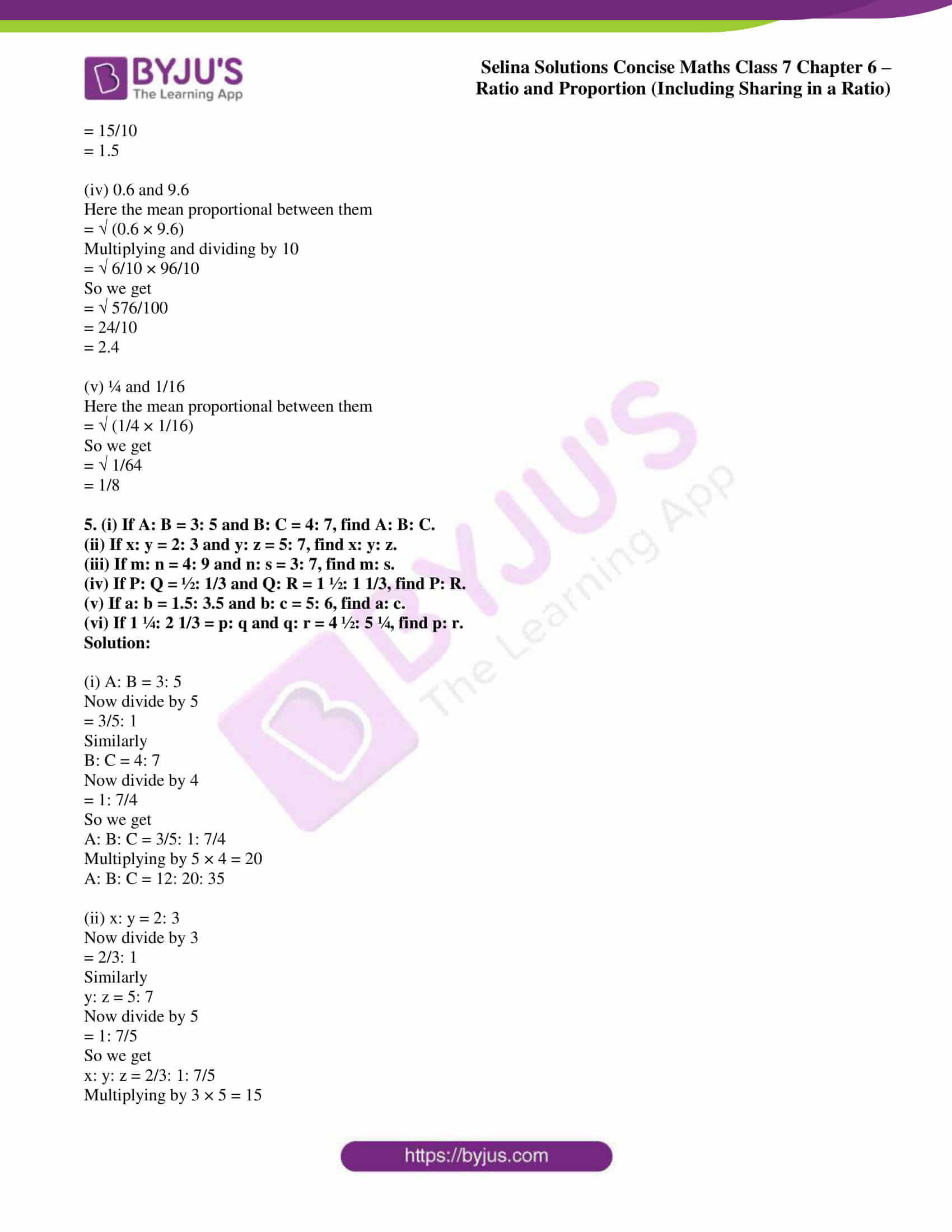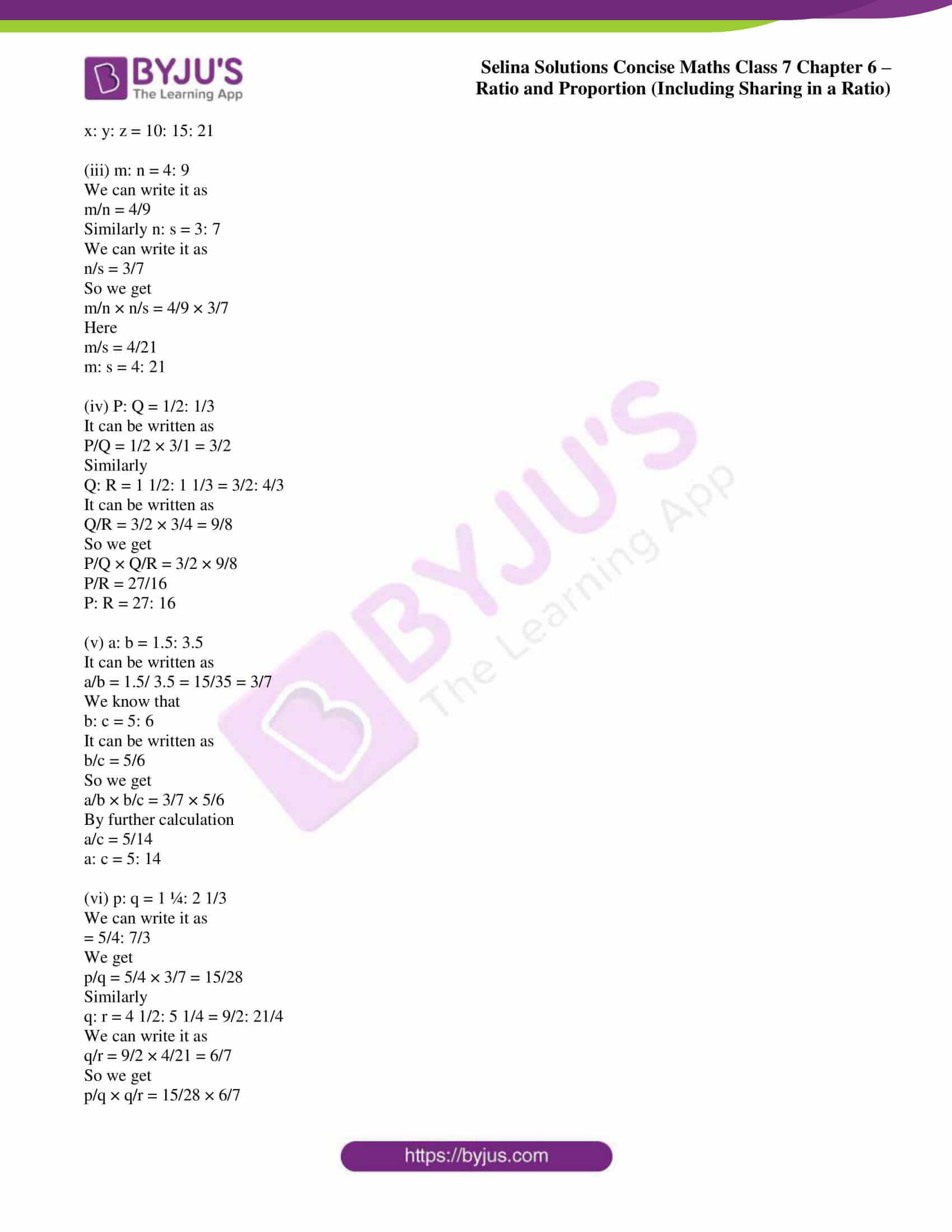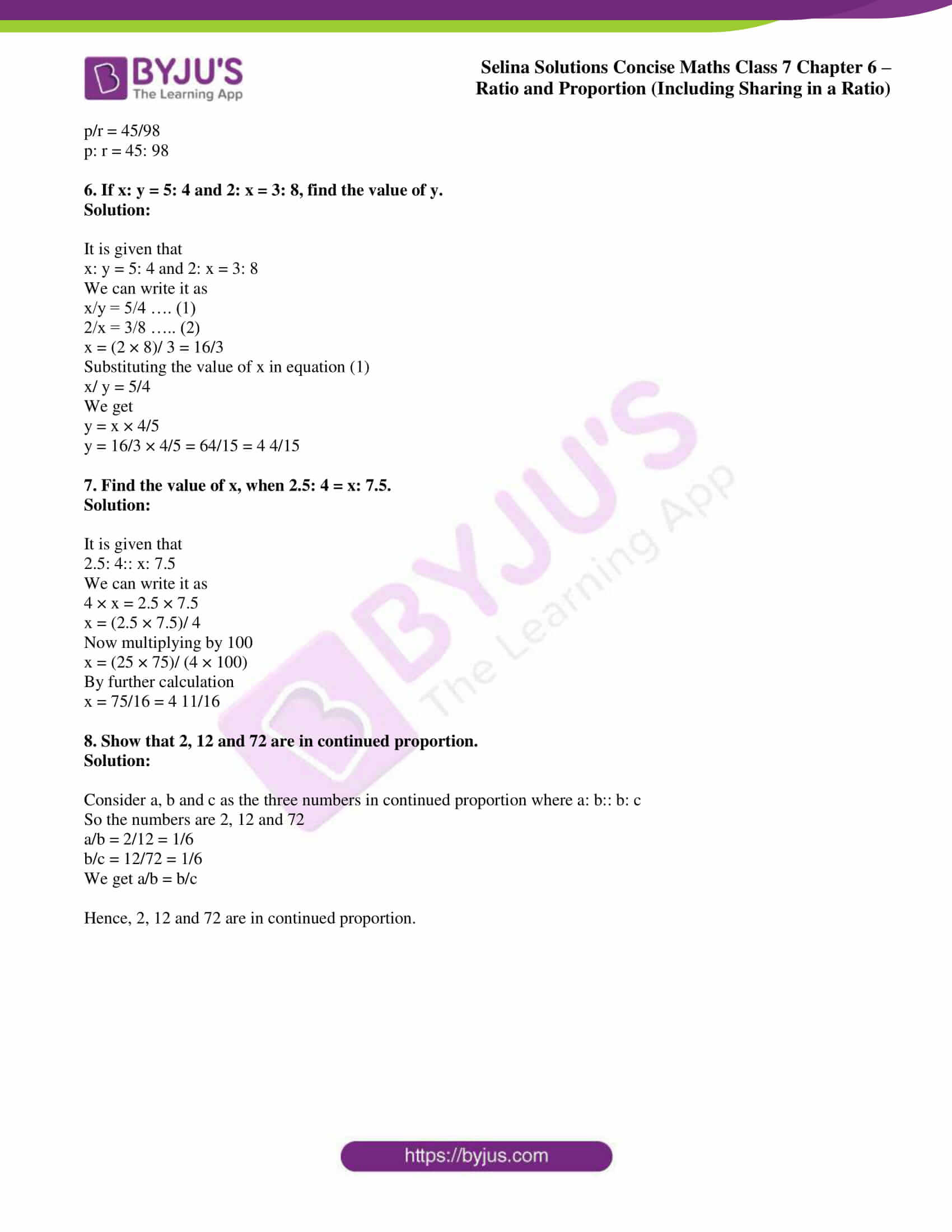### Access Selina Solutions Concise Maths Class 7 Chapter 6: Ratio and Proportion (Including Sharing in a Ratio) Exercise 6B

1. Check whether the following quantities form a proportion or not:

(i) 3x, 7x, 24 and 56

(ii) 0.8, 3, 2.4 and 9

(iii) 1 ½, 3 ¼, 4 1/2 and 9 ¾

(iv) 0.4, 0.5, 2.9 and 3.5

(v) 2 ½, 5 ½, 3.0 and 6.0

Solution:

(i) 3x, 7x, 24 and 56

If the quantities are in proportion

3x × 56 = 7x × 24

By further calculation

168x = 168x which is true

Therefore, 3x, 7x, 24 and 56 are in proportion.

(ii) 0.8, 3, 2.4 and 9

If the quantities are in proportion

0.8 × 9 = 3 × 2.4

By further calculation

7.2 = 7.2 which is true

Therefore, 0.8, 3, 2.4 and 9 are in proportion.

(iii) 1 ½, 3 ¼, 4 1/2 and 9 ¾

If the quantities are in proportion

1 ½ × 9 ¾ = 3 ¼ × 4 ½

By further calculation

3/2 × 39/4 = 13/4 × 9/2

117/8 = 117/8 which is true

Therefore, 1 ½, 3 ¼, 4 1/2 and 9 ¾ are in proportion.

(iv) 0.4, 0.5, 2.9 and 3.5

If the quantities are in proportion

0.4 × 3.5 = 0.5 × 2.9

By further calculation

1.40 = 1.45 which is not true

Therefore, 0.4, 0.5, 2.9 and 3.5 are not in proportion.

(v) 2 ½, 5 ½, 3.0 and 6.0

If the quantities are in proportion

2 ½ × 6.0 = 5 ½ × 3.0

By further calculation

5/2 × 6.0 = 11/2 × 3.0

30/2 = 33/2 which is not true

Therefore, 2 ½, 5 ½, 3.0 and 6.0 are not in proportion.

2. Find the fourth proportional of:

(i) 3, 12 and 4

(ii) 5, 9 and 45

(iii) 2.1, 1.5 and 8.4

(iv) 1/3, 2/5 and 8.4

(v) 4 hours 40 minutes, 1 hour 10 minutes and 16 hours

Solution:

(i) 3, 12 and 4

Here the 4th proportional = (12 × 4)/ 3 = 16

(ii) 5, 9 and 45

Here the 4th proportional = (9 × 45)/ 5 = 81

(iii) 2.1, 1.5 and 8.4

Here the 4th proportional = (1.5 × 8.4)/ 2.1 = 1.5 × 4 = 6.0

(iv) 1/3, 2/5 and 8.4

Here the 4th proportional = (2/5 × 8.4)/ 1/3

By further calculation

= 2/5 × 8.4 × 3/1

So we get

= (2 × 84 × 3)/ (5 × 10 × 1)

= 252/25

= 10.08

(v) 4 hours 40 minutes, 1 hour 10 minutes and 16 hours

It can be written as

4 hours 40 minutes = 4 × 60 + 40 = 240 + 40 = 280 minutes

1 hour 10 minutes = 1 × 60 + 10 = 60 + 10 = 70 minutes

16 hours = 16 × 60 = 960 minutes

So the fourth proportional = (70 × 960)/ 280 = 240 minutes

We get

= 240/60

= 4 hours

3. Find the third proportional of:

(i) 27 and 9

(ii) 2 m 40 cm and 40 cm

(iii) 1.8 and 0.6

(iv) 1/7 and 3/14

(v) 1.6 and 0.8

Solution:

(i) 27 and 9

Here the 3rd proportional = (9 × 9)/ 27 = 3

(ii) 2 m 40 cm and 40 cm

It can be written as

240 cm and 40 cm

Here the 3rd proportional = (40 × 40)/ 240 = 20/3 = 6 2/3 cm

(iii) 1.8 and 0.6

Here the 3rd proportional = (0.6 × 0.6)/ 1.8 = 0.36/ 1.8

Multiplying by 100

= 36/ 180

So we get

= 1/5

= 0.2

(iv) 1/7 and 3/14

Here the 3rd proportional = (3/14 × 3/14)/ 1/7

By further calculation

= 9/196 × 7/1

So we get

= 9/28

(v) 1.6 and 0.8

Here the 3rd proportional = (0.8 × 0.8)/ 1.6 = 0.64/1.6

By further calculation

= 64/160

= 2/5

= 0.4

4. Find the mean proportional between:

(i) 16 and 4

(ii) 3 and 27

(iii) 0.9 and 2.5

(iv) 0.6 and 9.6

(v) ¼ and 1/16

Solution:

(i) 16 and 4

Here the mean proportional between them

= √ (16 × 4)

By multiplication

= √ 64

= 8

(ii) 3 and 27

Here the mean proportional between them

= √ (3 × 27)

By multiplication

= √ 81

= 9

(iii) 0.9 and 2.5

Here the mean proportional between them

= √ (0.9 × 2.5)

Multiplying and dividing by 10

= √ (9/10 × 25/10)

So we get

= √ 225/100

= 15/10

= 1.5

(iv) 0.6 and 9.6

Here the mean proportional between them

= √ (0.6 × 9.6)

Multiplying and dividing by 10

= √ 6/10 × 96/10

So we get

= √ 576/100

= 24/10

= 2.4

(v) ¼ and 1/16

Here the mean proportional between them

= √ (1/4 × 1/16)

So we get

= √ 1/64

= 1/8

5. (i) If A: B = 3: 5 and B: C = 4: 7, find A: B: C.

(ii) If x: y = 2: 3 and y: z = 5: 7, find x: y: z.

(iii) If m: n = 4: 9 and n: s = 3: 7, find m: s.

(iv) If P: Q = ½: 1/3 and Q: R = 1 ½: 1 1/3, find P: R.

(v) If a: b = 1.5: 3.5 and b: c = 5: 6, find a: c.

(vi) If 1 ¼: 2 1/3 = p: q and q: r = 4 ½: 5 ¼, find p: r.

Solution:

(i) A: B = 3: 5

Now divide by 5

= 3/5: 1

Similarly

B: C = 4: 7

Now divide by 4

= 1: 7/4

So we get

A: B: C = 3/5: 1: 7/4

Multiplying by 5 × 4 = 20

A: B: C = 12: 20: 35

(ii) x: y = 2: 3

Now divide by 3

= 2/3: 1

Similarly

y: z = 5: 7

Now divide by 5

= 1: 7/5

So we get

x: y: z = 2/3: 1: 7/5

Multiplying by 3 × 5 = 15

x: y: z = 10: 15: 21

(iii) m: n = 4: 9

We can write it as

m/n = 4/9

Similarly n: s = 3: 7

We can write it as

n/s = 3/7

So we get

m/n × n/s = 4/9 × 3/7

Here

m/s = 4/21

m: s = 4: 21

(iv) P: Q = 1/2: 1/3

It can be written as

P/Q = 1/2 × 3/1 = 3/2

Similarly

Q: R = 1 1/2: 1 1/3 = 3/2: 4/3

It can be written as

Q/R = 3/2 × 3/4 = 9/8

So we get

P/Q × Q/R = 3/2 × 9/8

P/R = 27/16

P: R = 27: 16

(v) a: b = 1.5: 3.5

It can be written as

a/b = 1.5/ 3.5 = 15/35 = 3/7

We know that

b: c = 5: 6

It can be written as

b/c = 5/6

So we get

a/b × b/c = 3/7 × 5/6

By further calculation

a/c = 5/14

a: c = 5: 14

(vi) p: q = 1 ¼: 2 1/3

We can write it as

= 5/4: 7/3

We get

p/q = 5/4 × 3/7 = 15/28

Similarly

q: r = 4 1/2: 5 1/4 = 9/2: 21/4

We can write it as

q/r = 9/2 × 4/21 = 6/7

So we get

p/q × q/r = 15/28 × 6/7

p/r = 45/98

p: r = 45: 98

6. If x: y = 5: 4 and 2: x = 3: 8, find the value of y.

Solution:

It is given that

x: y = 5: 4 and 2: x = 3: 8

We can write it as

x/y = 5/4 …. (1)

2/x = 3/8 ….. (2)

x = (2 × 8)/ 3 = 16/3

Substituting the value of x in equation (1)

x/ y = 5/4

We get

y = x × 4/5

y = 16/3 × 4/5 = 64/15 = 4 4/15

7. Find the value of x, when 2.5: 4 = x: 7.5.

Solution:

It is given that

2.5: 4:: x: 7.5

We can write it as

4 × x = 2.5 × 7.5

x = (2.5 × 7.5)/ 4

Now multiplying by 100

x = (25 × 75)/ (4 × 100)

By further calculation

x = 75/16 = 4 11/16

8. Show that 2, 12 and 72 are in continued proportion.

Solution:

Consider a, b and c as the three numbers in continued proportion where a: b:: b: c

So the numbers are 2, 12 and 72

a/b = 2/12 = 1/6

b/c = 12/72 = 1/6

We get a/b = b/c

Hence, 2, 12 and 72 are in continued proportion.